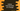# Python program to find out the perimeter of a triangle## Find the perimeter of a triangle in Python :

In this python programming tutorial, we will learn how to find out the perimeter of a triangle using_ user-provided_ values. The program will take the inputs from the user and print out the result.

## How to calculate the perimeter of a triangle :

The perimeter of a triangle is the sum of all of its sides. Let’s take a look at the below image :

The length of its sides is 4cm,5cm _and _4cm. So, the perimeter is 4 + 5 + 4 = 13cm. Our program will take the three sides as input from the user and print out the perimeter as output.

### Python program :

``````a = float(input("Enter the length of the first side in cm : "))
b = float(input("Enter the length of the second side in cm : "))
c = float(input("Enter the length of the third side in cm : "))

perimeter = a + b + c

print("The perimeter of the triangle is {} ".format(perimeter))``````

### Sample Output :

``````Enter the length of the first side in cm : 5
Enter the length of the second side in cm : 4
Enter the length of the third side in cm : 4
The perimeter of the triangle is 13.0

Enter the length of the first side in cm : 40
Enter the length of the second side in cm : 90
Enter the length of the third side in cm : 100
The perimeter of the triangle is 230.0

Enter the length of the first side in cm : 3
Enter the length of the second side in cm : 3
Enter the length of the third side in cm : 3
The perimeter of the triangle is 9.0``````